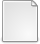# The Matrix Unwinding Function, with an Application to Computing the Matrix Exponential

Aprahamian, Mary and Higham, Nicholas J. (2014) The Matrix Unwinding Function, with an Application to Computing the Matrix Exponential. SIAM Journal on Matrix Analysis and Applications, 35 (1). pp. 88-109. ISSN 1095-7162PDF 130920137.pdf Download (313kB)Other unwinding.zip Download (2kB)
Official URL: http://epubs.siam.org/journal/sjmael

## Abstract

A new matrix function corresponding to the scalar unwinding number of Corless, Hare, and Jeffrey is introduced. This matrix unwinding function, $\mathcal{U}$, is shown to be a valuable tool for deriving identities involving the matrix logarithm and fractional matrix powers, revealing, for example, the precise relation between $\log A^\alpha$ and $\alpha \log A$. The unwinding function is also shown to be closely connected with the matrix sign function. An algorithm for computing the unwinding function based on the Schur--Parlett method with a special reordering is proposed. It is shown that matrix argument reduction using the function $\mathrm{mod}(A) = A-2\pi i\, \mathcal{U}(A)$, which has eigenvalues with imaginary parts in the interval $(-\pi,\pi]$ and for which $\e^A = \e^{\mathrm{mod}(A)}$, can give significant computational savings in the evaluation of the exponential by scaling and squaring algorithms.

Item Type: Article matrix unwinding function, unwinding number, matrix logarithm, matrix power, matrix exponential, argument reduction MSC 2010, the AMS's Mathematics Subject Classification > 15 Linear and multilinear algebra; matrix theoryMSC 2010, the AMS's Mathematics Subject Classification > 65 Numerical analysis Nick Higham 01 Feb 2014 20 Oct 2017 14:13 http://eprints.maths.manchester.ac.uk/id/eprint/2094

### Available Versions of this ItemView Item